Courses

# Structures

## 25 Questions MCQ Test GATE Civil Engineering (CE) 2022 Mock Test Series | Structures

Description
This mock test of Structures for GATE helps you for every GATE entrance exam. This contains 25 Multiple Choice Questions for GATE Structures (mcq) to study with solutions a complete question bank. The solved questions answers in this Structures quiz give you a good mix of easy questions and tough questions. GATE students definitely take this Structures exercise for a better result in the exam. You can find other Structures extra questions, long questions & short questions for GATE on EduRev as well by searching above.
QUESTION: 1

### Select the correct option, The given structure is,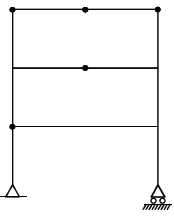Solution: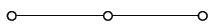Three consecutive hinges form a mechanism.

QUESTION: 2

### Find the degree of indeterminacy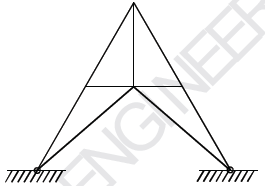Solution: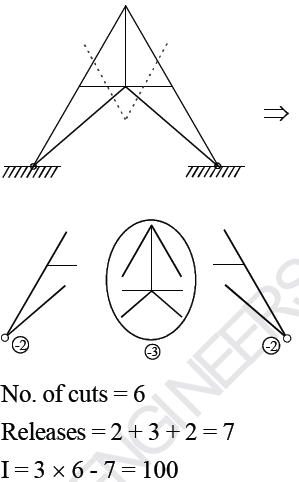QUESTION: 3

### Find the external indeterminacy,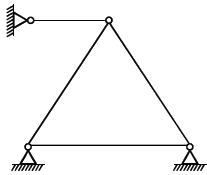Solution: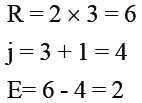QUESTION: 4

What will be the kinematic Indeterminacy for the structure,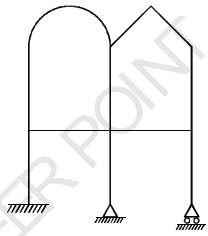Solution: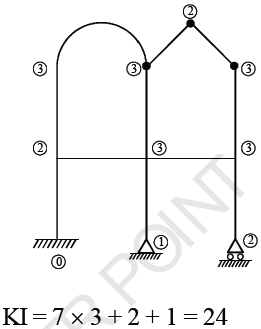QUESTION: 5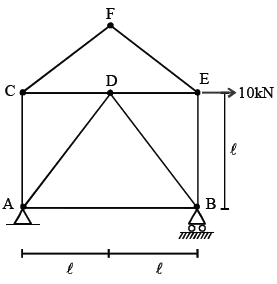Find force in AD.

Solution: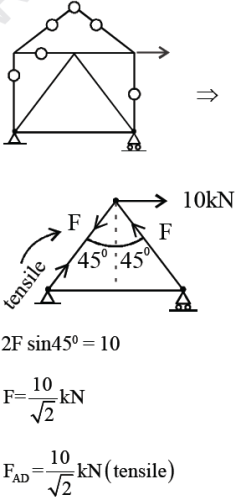QUESTION: 6

If number, CD has been fabricated 10 mm short, by how much will point C deflect vertically?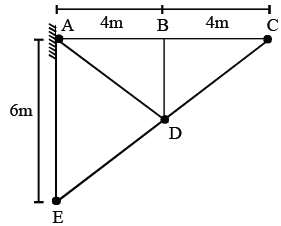Solution: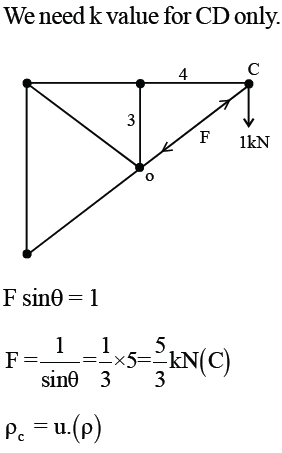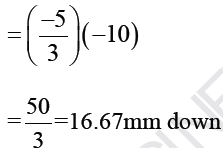QUESTION: 7

What is Shear force at section X-X in three hinged frame shown,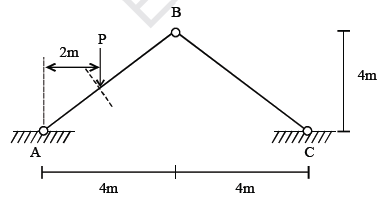Solution: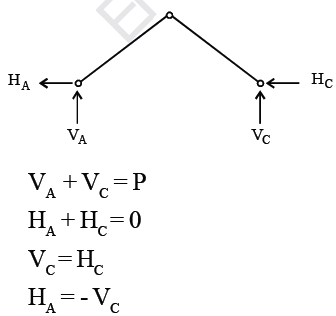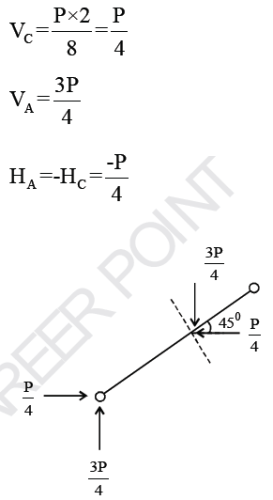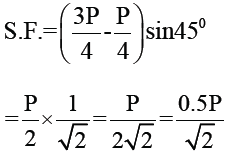QUESTION: 8

Assertion: In analysis of rigid frames, usual practice is to consider strain energy due to flexure only.

Reason: Strain Energy due to axial & shear force are usually quite small compared to that of flexure.

Solution:
QUESTION: 9

Find the strain energy stored in beam,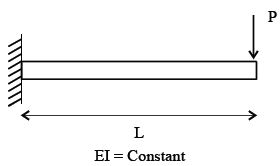Solution: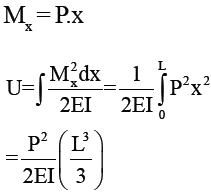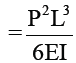QUESTION: 10

What is horizontal deflection at free end C.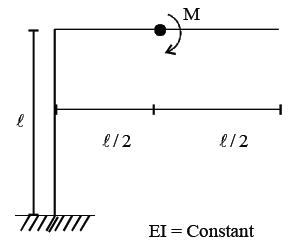Solution: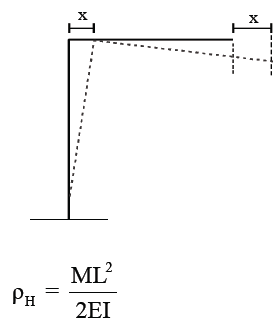QUESTION: 11

A single bay frame (Single storey) has hinged left support & fixed right support. It is loaded with udl on beam.
Find the correct statement.

Solution:

Weaker column is left column, so sway towards left.

QUESTION: 12

Find the fixed end moments,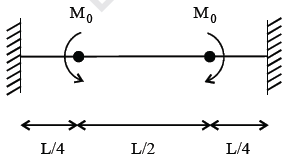Solution: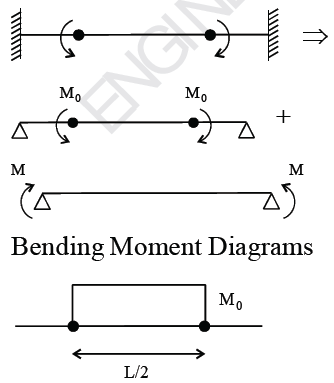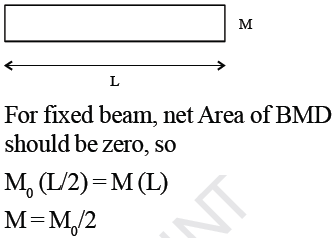QUESTION: 13

A uniform beam of length 2L & flexural rigidity EI is fixed at both the ends. What is moment required for unit rotation at centre of span.

Solution: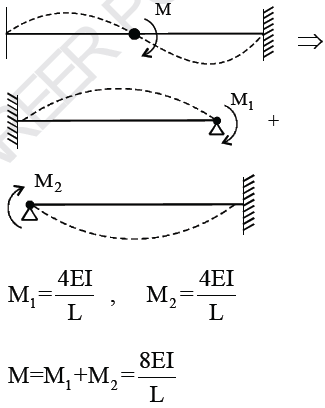QUESTION: 14

Propped cantilever AB carries a udl of q/unit length.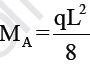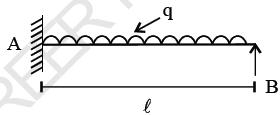Solution: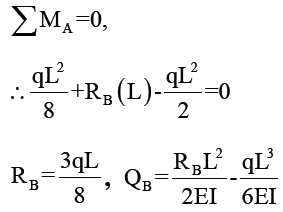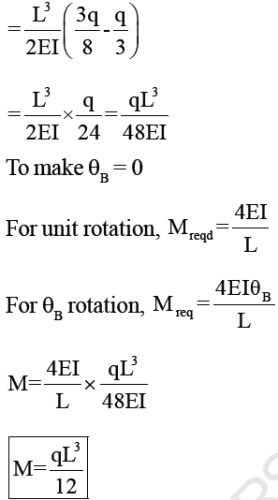QUESTION: 15

Fixed beam AB with central hinge C, is built of two components. AC is rigid and CB has a moment of inertia I. When support A yields and rotates by θ,what is moment at B?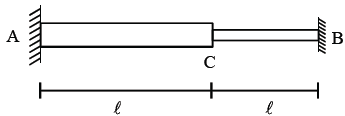Solution: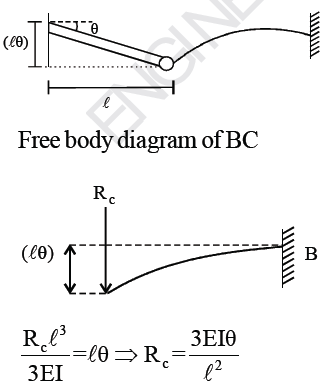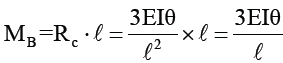QUESTION: 16

Find the relative displacement of A & B.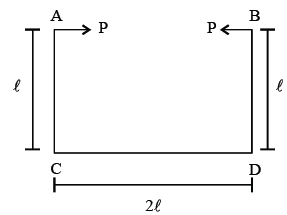EI = constant

Solution: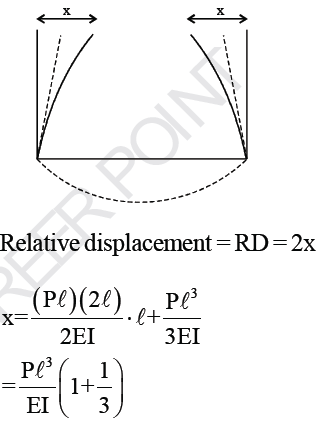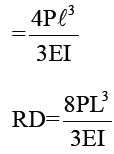QUESTION: 17

For the semicircular arch shown in figure, moment of 30 t-cm applied at B produces a displacement of 0.5 cm at A. If a concentrated load of 10t is applied at A, the rotation at B will be

Solution: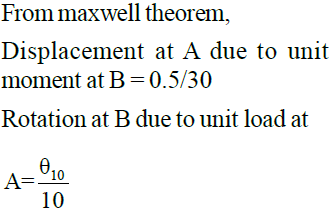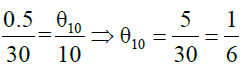QUESTION: 18

For propped cantilever, influence line for reaction at end B is given by y = f(x)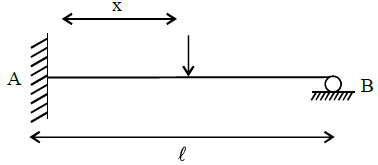The influence line ordinate (y') for moment at A is given by

Solution: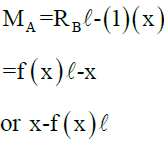QUESTION: 19

What is ordinate of influence line at B for reaction R0 in figure below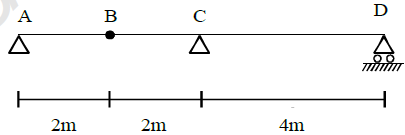Solution: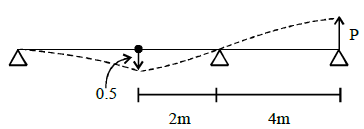QUESTION: 20

Find the flexibility coefficient f12 for continous beam shown,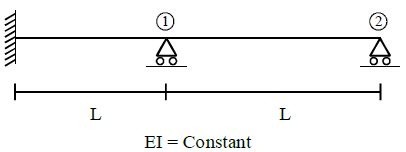Solution: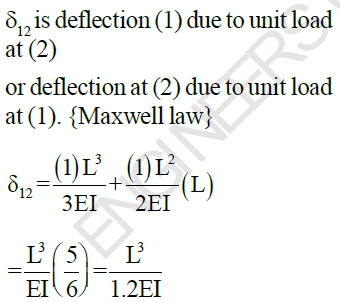*Answer can only contain numeric values
QUESTION: 21

Find the moment developed at the fixed end D. Assume the beam to have infinite rigidity. The end moment at D is ____ kNm.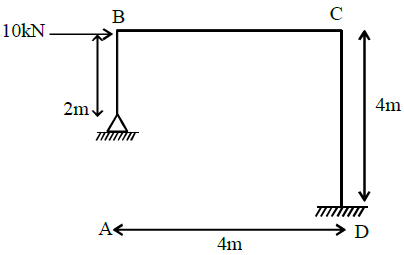Solution:

Due to Δ sway,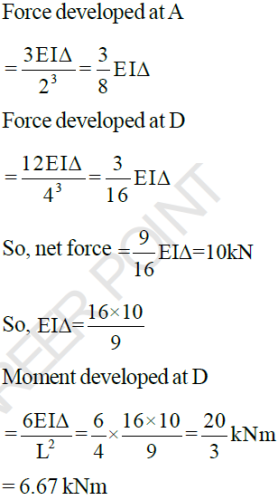QUESTION: 22

Moment Distribution factors for joints B & C are respectively.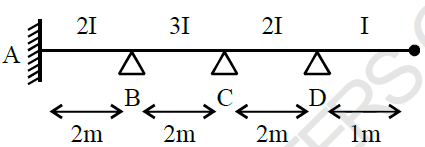Solution: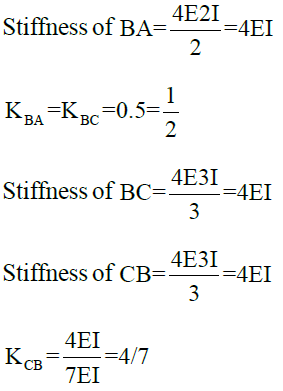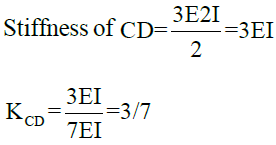QUESTION: 23

Find the reaction at B due to the given loading in the beam.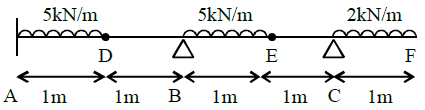Solution: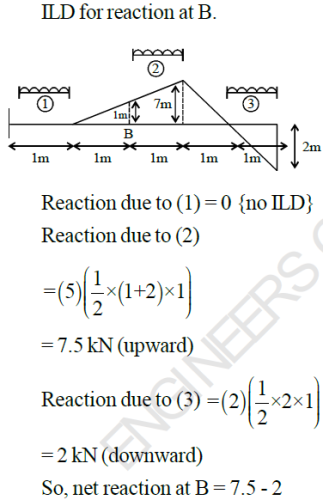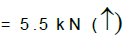QUESTION: 24

Which of the following is ILD for force in DG?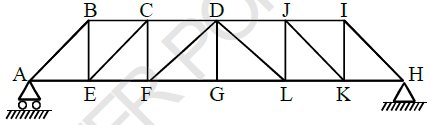Solution:

Member DG will experience force only where is some load at joint G.
This will happen when unit load is in panel FG or GL.

QUESTION: 25

Find the bending moment of at the section X - X. In the given structure. All joints are rigid.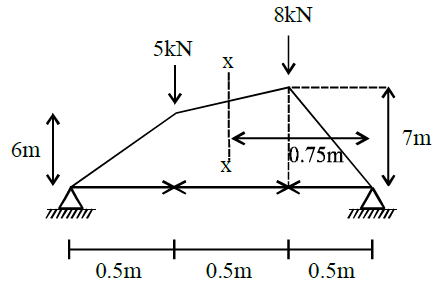Solution: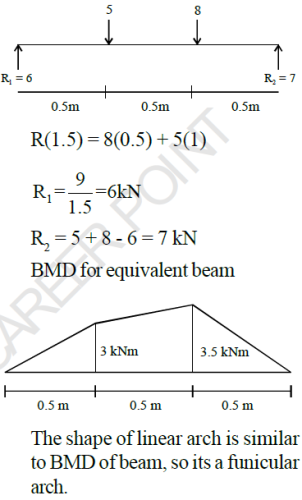Hence BM = 0 everywhere